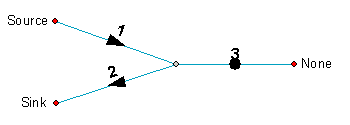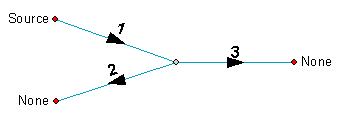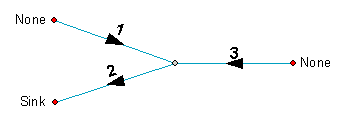English

# FAQ: How can edges have indeterminate flow after setting flow direction with multiple sources and sinks?

## Question

How can edges have indeterminate flow after setting flow direction with multiple sources and sinks?

If a geometric network has multiple sources and sinks connected to each other, the flow direction for some edges is set to indeterminate flow.

Multiple sources and sinks usually cause conflicting flow direction, which yields indeterminate flow.

The example below illustrates this by examining how flow direction works.

Consider a geometric network with the sources and sinks positioned as below:The flow direction is set as shown above.

To explain why edge 3 has indeterminate flow, let's consider the case where only the source is present. The flow direction will be set as below:Note edge 3 is flowing to the right. The example below illustrates what happens if only the sink is present:Edge 3 is now flowing to the left, and is the opposite of the previous example. This is a conflict.

For each edge, if the flow direction is in agreement between both the source-only and sink-only cases, the flow direction is set to that direction, as with edges 1 and 2. If there is a conflict, as there is with edge 3, the flow direction is set to indeterminate since there are two possible outcomes.